# Microsoft Azure – Tracking Memory Utilization of Azure VM using KQL Log Query

• Last Updated : 06 Dec, 2021

In this article, we will look into the process of finding the Minimum, Average, and Maximum Memory Utilization of all Azure Virtual Machines using KQL Log Query. Here we are Perf KQL Counter Operator which is used to find Azure Virtual Machines performance from collected data logs.

### Implementation:

Follow the below steps to Run the Log Queries.

Step 2: Search/Go to Log Analytics Workspace and select your Log Analytics Workspace. Create one if you don’t have any and enable agent configurations.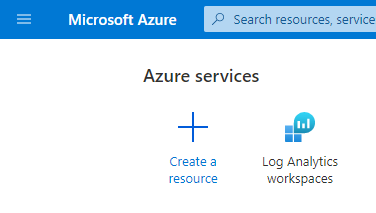• Then, from the left menu navigate to General >> Logs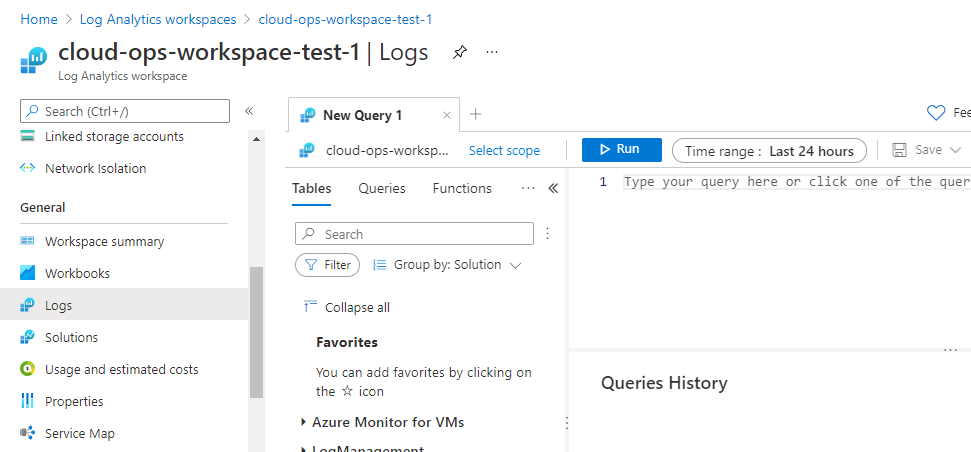• Now, Paste the below Queries to get the log data.
• Before Running the Query understand the Query Syntax.

### Explanation for all the below examples :

Here, Perf represents performance, this is an operator which is used to fetch the performance logs of Azure Compute resources like Azure VMs, SQL Servers, Disk Storage,…etc.

We are using the ObjectName == “Memory” and CounterName == “% Committed Bytes In Use” (To calculate the Windows Metrics) and CounterName == “% Used Memory” (To calculate the Linux Metrics) these conditions filters the memory performance components of Windows and Linux VMs using a where keyword.

TimeGenerated > ago(7d) or TimeGenerated > ago(5h) helps in filter the logs collected within that timespan.

With the summarize keyword we are rendering the required data in the form of a table chart.

### Find the following KQL Queries for Analysing Memory Performance of Azure VMs.

Example: To find Minimum Memory Utilization of Azure Virtual Machines for last 7 days

```Perf
| where ObjectName == "Memory"
| where CounterName == "% Used Memory" or CounterName == "% Committed Bytes In Use"
| where TimeGenerated > ago(7d)
| summarize MIN_MEMORY = min(CounterValue) by Computer, _ResourceId```

Output: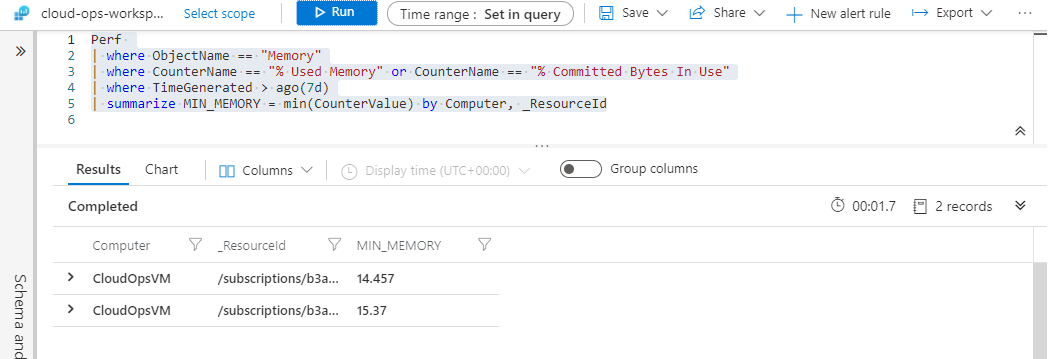Example: To find Minimum Memory Utilization of Azure Virtual Machines for the last 5 hours

```Perf
| where ObjectName == "Memory"
| where CounterName == "% Used Memory" or CounterName == "% Committed Bytes In Use"
| where TimeGenerated > ago(5h)
| summarize MIN_MEMORY = min(CounterValue) by Computer, _ResourceId```

Output: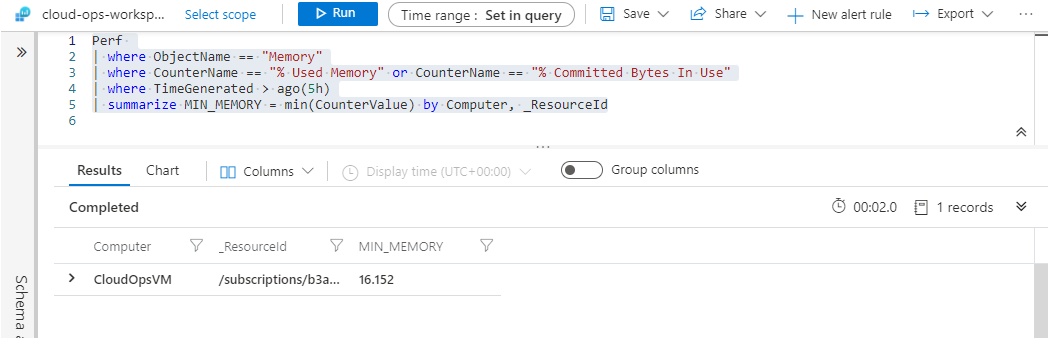Example 1: To find Average Memory Utilization of Azure Virtual Machines for the last 7 days.

```Perf
| where ObjectName == "Memory"
| where CounterName == "% Used Memory" or CounterName == "% Committed Bytes In Use"
| where TimeGenerated > ago(7d)
| summarize AVG_MEMOERY= avg(CounterValue) by Computer, _ResourceId```

Output: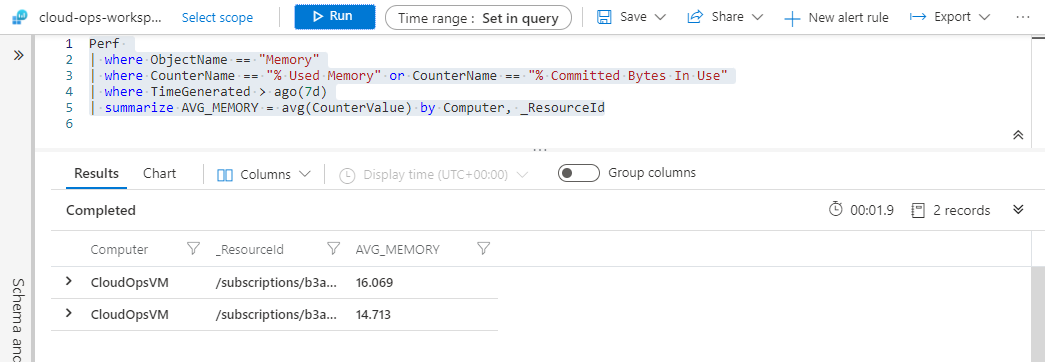Example 2: To find Average Memory Utilization of Azure Virtual Machines for the last 5 hours

```Perf
| where ObjectName == "Memory"
| where CounterName == "% Used Memory" or CounterName == "% Committed Bytes In Use"
| where TimeGenerated > ago(7d)
| summarize AVG_MEMOERY= avg(CounterValue) by Computer, _ResourceId```

Output: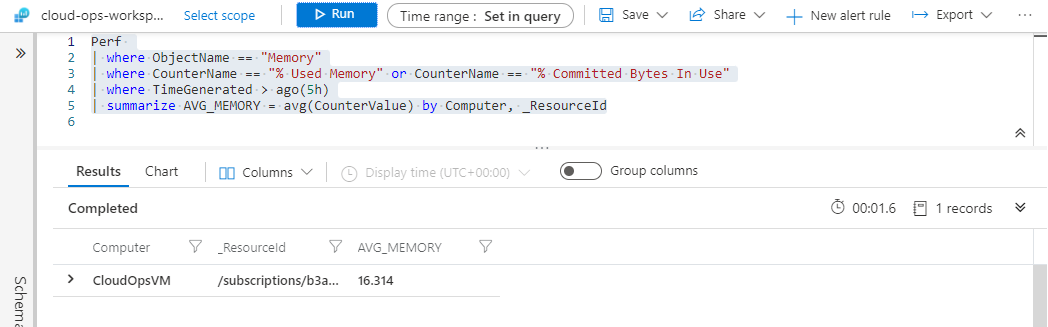Example 3: To find Maximum Memory Utilization of Azure Virtual Machines for the last 7 days

```Perf
| where ObjectName == "Memory"
| where CounterName == "% Used Memory" or CounterName == "% Committed Bytes In Use"
| where TimeGenerated > ago(7d)
| summarize MAX_MEMORY = max(CounterValue) by Computer,  _ResourceId```

Output: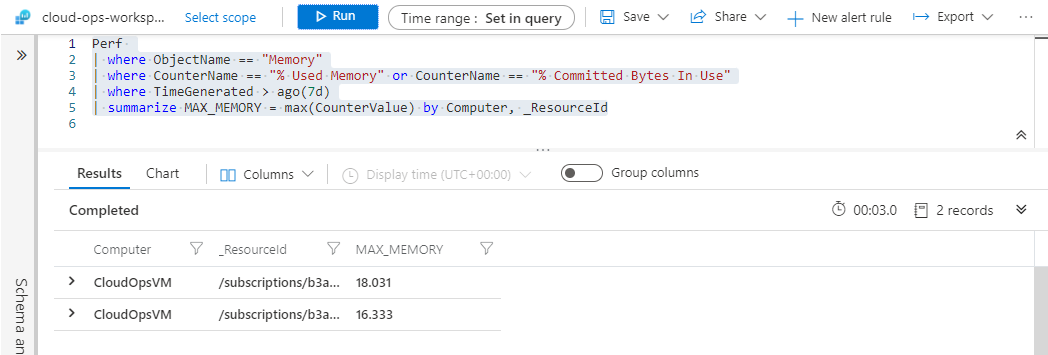Example 4: To find Maximum Memory Utilization of Azure Virtual Machines for last 5 hours

```Perf
| where ObjectName == "Memory"
| where CounterName == "% Used Memory" or CounterName == "% Committed Bytes In Use"
| where TimeGenerated > ago(5h)
| summarize  MAX_MEMORY = max(CounterValue) by Computer,  _ResourceId```

Output: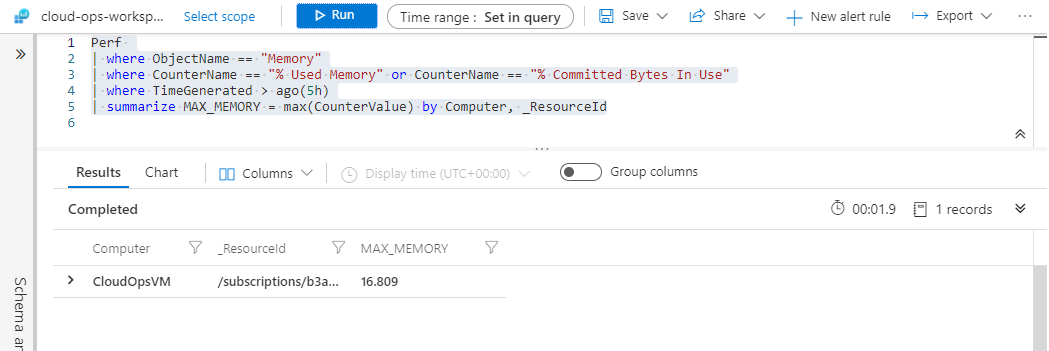Example 5: To analyze VM Memory Utilization of Minimum, Average and Maximum for last 7 days in a single table chart.

```Perf
| where ObjectName == "Memory"
| where CounterName == "% Used Memory" or CounterName == "% Committed Bytes In Use"
| where TimeGenerated > ago(7d)
| summarize MIN_MEMORY = min(CounterValue), AVG_MEMOERY= avg(CounterValue), MAX_MEMORY = max(CounterValue) by Computer,  _ResourceId```

Output: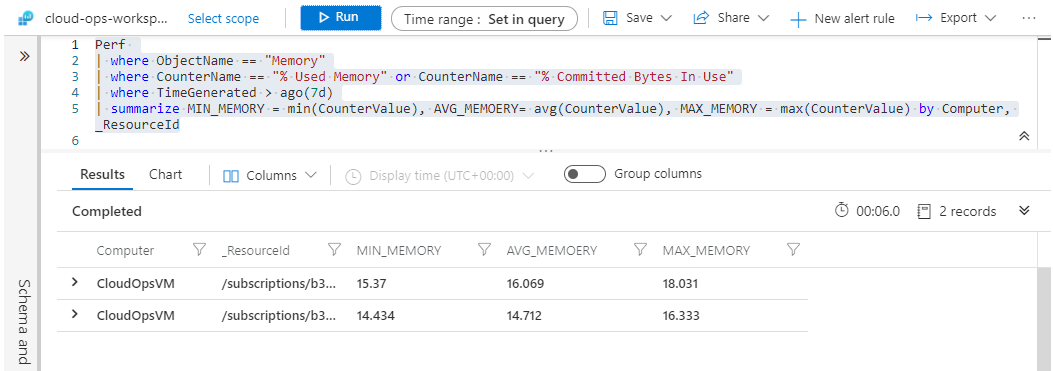Example 6: To analyze VM Memory Utilization of Minimum, Average and Maximum for last 5 hours in a single table chart.

```Perf
| where ObjectName == "Memory"
| where CounterName == "% Used Memory" or CounterName == "% Committed Bytes In Use"
| where TimeGenerated > ago(5h)
| summarize MIN_MEMORY = min(CounterValue), AVG_MEMOERY= avg(CounterValue), MAX_MEMORY = max(CounterValue) by Computer,  _ResourceId```

Output: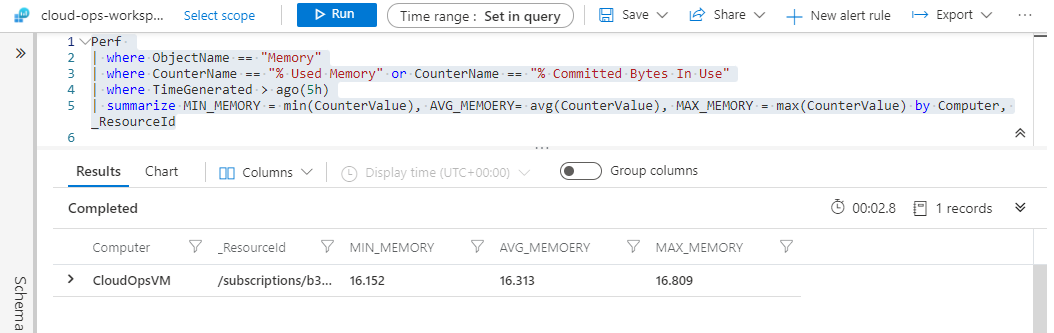That’s it, you are done.

My Personal Notes arrow_drop_up